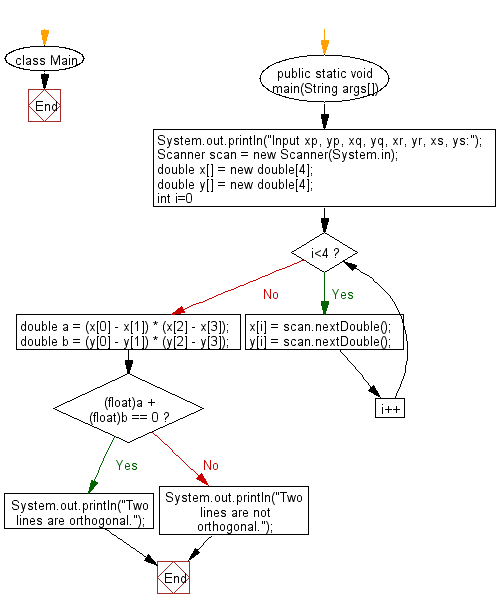# Java Exercises: Test whether AB and CD are orthogonal or not

## Java Basic: Exercise-235 with Solution

There are four different points on a plane, P(xp,yp), Q(xq, yq), R(xr, yr) and S(xs, ys).
Write a Java program to test whether AB and CD are orthogonal or not.

Input:
xp,yp, xq, yq, xr, yr, xs and ys are -100 to 100 respectively and each value can be up to 5 digits after the decimal point It is given as a real number including the number of.
Output: Yes or No.

Sample Solution:

Java Code:

`````` import java.util.*;
import static java.lang.Math.*;

class Main{
public static void main(String args[]){
System.out.println("Input xp, yp, xq, yq, xr, yr, xs, ys:");
Scanner scan = new Scanner(System.in);
double x[] = new double;
double y[] = new double;
for(int i=0;i<4;i++){
x[i] = scan.nextDouble();
y[i] = scan.nextDouble();
}
double a = (x - x) * (x - x);
double b = (y - y) * (y - y);
if((float)a + (float)b == 0)
System.out.println("Two lines are orthogonal.");
else
System.out.println("Two lines are not orthogonal.");
}
}
```
```

Sample Output:

```Input xp, yp, xq, yq, xr, yr, xs, ys:
3.5 4.5 2.5 -1.5 3.5 1.0 0.0 4.5
Two lines are not orthogonal.
```

Pictorial Presentation:Flowchart:Java Code Editor:

What is the difficulty level of this exercise?

﻿

## Java: Tips of the Day

Parsing dates:

```import java.io.*;
import java.util.*;
import java.text.*;

String s = "2001/09/23 14:39";

SimpleDateFormat formatter = new SimpleDateFormat ("yyyy/MM/dd H:mm");
Date d = formatter.parse(s, new ParsePosition(0));
```